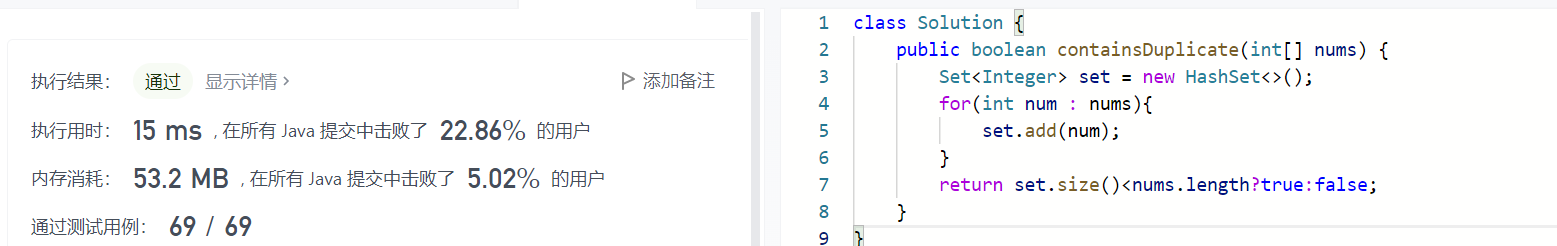# 腾讯五十题 No.39 存在重复元素

Set实现Map实现

``````class Solution {
public boolean containsDuplicate(int[] nums) {
int len = nums.length;
Map<Integer,Integer> map = new HashMap<>(len);
for(int i = 0;i<len;i++){
if(map.containsKey(nums[i])){
return true;
}
map.put(nums[i],i);
}
return false;
}
}
``````

### 推荐这些文章：

// 项目场景说明： 对于运单的常跑进行显示，前端页面显示5个常跑地址 public class MapSortUtils {
/**
* @冒泡排序（降序）
* @param map
* @return
*/
public static LinkedHashMap<String,Integer> mapSort( Map<String,Integer> map){

LeetCode 219. 存在重复元素 II

219. 存在重复元素 II
Solution

class Solution {
public boolean containsNearbyDuplicate(int[] nums, int k) {
Map<Integer, Integer> mp = new HashMap<>();
int len = nums.length;
for (int i = 0; i < len; i++) {
if...

Java统计List集合中每个元素出现的次数

public static Map<String,Integer> frequencyOfListElements( List items ) {
if (items == null || items.size() == 0) return null;
Map<String, Integer> map = new HashMap<String, Integer>();
for (String temp : items) {
Integer count = map.get(temp);
map.put(temp, (count == null) ? 1 : ...

if判断条件过多,利用Map,让代码表达更清晰

int a = 1;
if(a ==1 ||  a == 3 || a == 4 || a == 8 || a == 11){
}
=====================================
Map<Integer,Boolean> map = new HashMap();
map.put( 1, true);
map.put( 3, true);
map.put( 4, true);
map.put( 8, true);
map.put( 11, true);
int a = 1;
if(map.get( a )){
}
...

1.如果存在，返回其值
2.如果不存在，利用map.set()函数将元素及其索引添加到map中

var findRepeatNumber = function(nums) {
let map=new Map();
for(let i=0;i<...

LeetCode 1748. Sum of Unique Elements

LeetCode 1748. Sum of Unique Elements (唯一元素的和)

https://leetcode-cn.com/problems/sum-of-unique-elements/

1 <= nums.length <= 100
1 <= nums[i] <= 100

JAVA中对List<map<String,Object>>根据map某个key值从小到大顺序进行排序

public static void main(String[] args) {
List<Map<String, Object>> list = new ArrayList<Map<String, Object>>();
Map<String, Object> map1 = new HashMap<String, Object>();
map1.put("name", "p");
map1.put("周", "10");
Map<String, Objec...

532--数组中的 k-diff 数对（技巧+感悟）

0 <= i < j < nums.length
|nums[i] - nums[j]| == k

package Com.Xu.DoublePointer;

import java.util.HashMap;
import java.u...

Java 获取重复的值 并统计总数

public static void main(String[] args) {
List l =new ArrayList();
System.out.println(l);
Map<String, Integ...

217. 存在重复元素

示例 1:
输入: [1,2,3,1]　　输出: true
示例 2:
输入: [1,2,3,4]　　输出: false

THE END